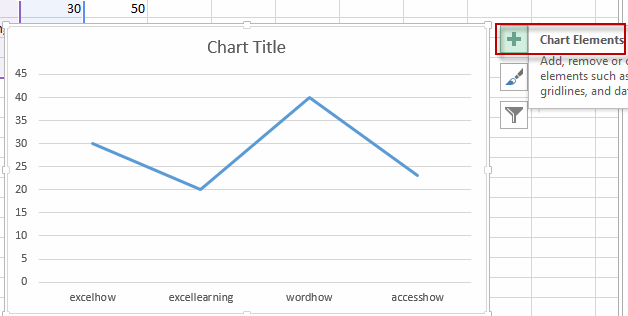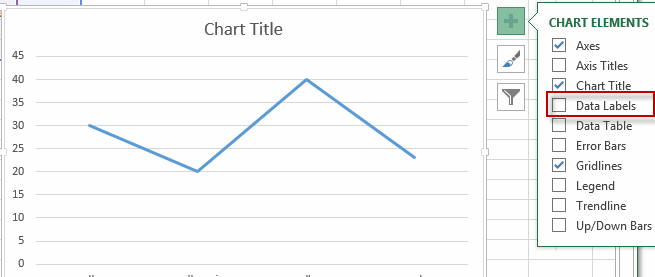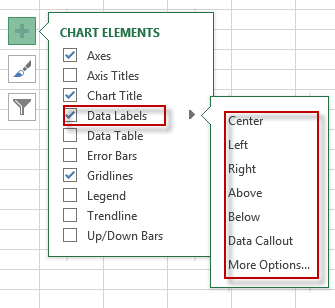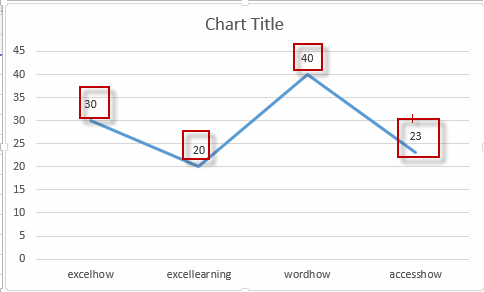# Add Data Labels in a Chart

This post will guide you how to add data labels into a newly created excel chart. How do I add or move data labels to a chart in Excel 2013/2016.How to add data labels to a chart in excel 2010 or 2007.

## Add Data Labels in a Chart

When you created a chart in your current worksheet, the data label are not shown in the chart by default. So if you want to add data labels in the chart, how to achieve it. Just do the following steps:

For Excel 2013/2016:

#1 click the chart that you just created, and click Chart Elements button in the upper right corner next to the chart.#2 check Data Labels option from the popup list.#3 click Arrow to choose an option to change the location of data labels.#4 let’s see the resultFor Excel 2007 or 2010:

#1 click on the chart that you just created.

#2 go to Layout tab, click Data Labels command under Chart Tools group.

#3 choose the position of data labels, such as: Center or Inside End…so on.

Related Posts

Cap percentage values between 0 and 100

This article will talk about how to cap the percentage values between 0% and 100% in Microsoft Excel Spreadsheet or Google Sheets. If you are a newbie on Excel or google Sheets, you may be able to do this by ...

Calculate Cap Percentages to Specific Value

This article will talk about how to limit the cap percentage of a given amount to a given value in Microsoft Excel Spreadsheet or Google Sheets. If you are a newbie on Excel or google Sheets, you may be able ...

Calculate Win Loss Tie

Suppose you got a task to calculate the win, loss, and tie totals; what would you do? If you are new to Ms Excel and don't have enough experience with it, then you might do this task manually but let ...

Calculate Years Between Dates In Ms Excel

If you are an avid Ms Excel user, then you might have come across a task in which you needed to calculate the years between the dates; you might take it easy and do this task manually, which is also ...

Calculate Number of Hours between Two Times

Calculating the difference between two times might be a valuable statistic for subsequent computations or averages, whether you're producing a time sheet for staff or recording personal exercises. While Excel has a plethora of complex functions, including date and time ...

Calculate Loan Interest in Given Year

When you borrow money, you are supposed to repay it gradually. Lenders, on the other hand, want to be compensated for their services and the risk they incur by lending you money. That is, you will not just repay the ...

Calculate Interest Rate for Loan

The interest rate is the fee charged by a lender to a borrower and is expressed as a percentage of the principal—the lent amount. The interest rate on a loan is often expressed as an annual percentage rate, abbreviated as ...

Calculate Interest for Given Period

Using the IPMT function in Excel, we can compute the interest payment on any loan. This step-by-step tutorial will guide Excel users of all skill levels through the process to calculate interest for given period. Finally, the formula: =IPMT(B3/12,1,B5,-B2) The ...

How To Use Excel GCD Function

This post will guide you how to use Excel GCD function with syntax and examples in Microsoft excel. Description The Excel GCD function Returns the greatest common divisor of two or more integers. So you can use the GCD function ...

Calculate A Ratio From Two Numbers In Excel

In elementary mathematics, a ratio is a connection or comparison between two or more integers. For example, ratios are often expressed as ":" to demonstrate the relationship between two numbers. You would think that manually calculating a ratio from two ...

Sidebar